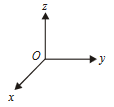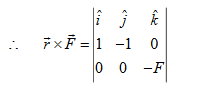#### A force ofacts on O, the origin of the coordinate system. The torque about the pointis :Option 1)Option 2)Option 3)Option 4)As we learnt in

Torque -- wherein

This can be calculated by using either= perpendicular distance from origin to the line of force.= component of force perpendicular to line joining force.Option 1)This is an incorrect option.

Option 2)This is an incorrect option.

Option 3)This is an incorrect option.

Option 4)This is the correct option.

##### Posted by